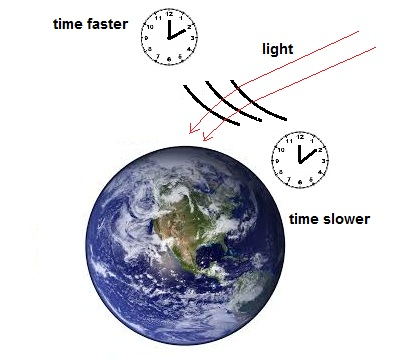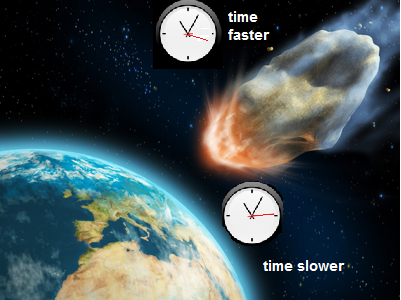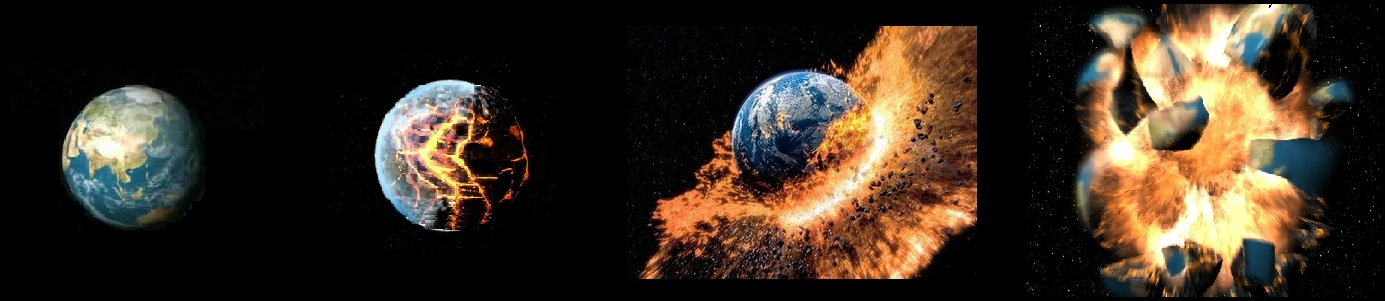The search for  new physics.

If gravitational time dilation is real, why has the earth not torn apart?

Doug Marett, 2013

Introduction:

It is common knowledge that Einstein’s theory of relativity calls for the passage of time to be a relative phenomenon that depends on the perspective of the observer, namely their rate of motion or their position in a gravitational gradient. The former phenomenon is known as velocity time dilation, and the latter is known as gravitational time dilation. The theory actually calls for the rate of real time to be different for various observers, such that no such thing as “absolute time” can exist. Experimental evidence has shown that atomic clocks will actually speed up as they go to higher altitudes, appearing to confirm his predictions. However, earlier scientific theories, such as the work of Lorentz, Larmor, and Poincare, had suggested that such a phenomenon might occur for far more mundane reasons, namely as an illusion due to the finite speed of light and the dependence of clocks on the same. The question addressed herein is not whether clocks count faster or slower in a gravitational gradient, but rather if the increase or decrease in the apparent passage of time is an illusion.

Einstein’s 1911 Paper:

In Einstein’s paper “On the Influence of Gravitation on the Propagation of Light” (1911) he argues that light travelling at an oblique angle bends downward as it approaches the surface of a body like the earth, since time becomes progressively slower as one goes lower in a gravitational potential. The mechanism is as follows:

1)      As light wavefronts approach the surface, the lower edge of the wavefront slows down with respect to the upper edge, since time closer to the gravitational body is counting slower.

2)      This progressive bending of the wavefront turns the angle of the approaching light progressively down.

3)      The speed of light when measured by clocks in their local time is still C, but if synchronized clocks are used, then the speed of light would be measured as progressively slower as one approaches the surface.The fundamental issues around Einstein’s treatment have already been dealt with in our previous paper “Light Propagation in a Gravitational Field.” We want to focus here on how light behaves with respect to local time. In order for light to bend as Einstein claims due to gravitational time dilation, the light remains in the same "co-relational present” along the wavefront. In other words, no part of the wavefront will advance or recede in local time in relation to any other part of the same wavefront.  It is because the entire wavefront remains in the same co-relational present as it moves that causes the bending. Thus if the wavefront at the top is at 12:10AM local time, it is also at 12:10AM local time at the bottom.  Why is this important? Because it helps define how Einstein interprets time and the connectivity of objects which are large enough to straddle many local time frames. The phenomenon should also apply to moving massive particles as has been suggested here. If we extrapolate that matter should be affected in the same way as light, then we would similarly have to believe that if a large asteroid were to enter the earth’s atmosphere, its surface would bend to accommodate the difference is the rate of local time between its upper and lower surface, since as time counts slower, the velocity of the lower edge must travel slower than the upper edge of the interconnected mass. Velocity = distance/time, so if the unit of time become longer then the velocity becomes smaller. Of course this difference in the rate of time across the asteroid is extremely small, so any bending might be imperceptible. The situation is different than that of a wavefront since it is impossible for the entire body of the asteroid to remain in the same co-relational present if time dilation is in operation.  However, a given point x on the asteroid would tend to distort from its original shape to preserve the co-relational progression of the event in local time. This also presents the paradox that a single object would then exist partly in the past, present, and future.

Asteroid approaching the earth at an oblique angle – the body of the asteroid should

bend slightly toward the earth’s surface due to gravitational time dilation.What if we consider a much larger object that is bending for a much longer time? The obvious choice would be the earth itself.  According to Einstein’s theory, the surface of the earth where we are standing should experience a local time that is heading into the future at a rate somewhat faster than the core 6371 km below our feet. Since the earth is a substantially solid object through most of its radius (excluding the outer core), it should experience rigid body rotation for the majority of its mass. The difference in the rate of time from the center to the surface would however imply that the angular velocity should actually increase as we approach the surface. So is this velocity difference significant?  What would this value be?

The gravitational time dilation difference from the surface to the core is t’ = t*(1+ GM/(2Rc^2) )

GM = 3.9860E14           R = 6371000 (at the equator)   C = ~3 E8

Thus GM/(2Rc^2) = 3.481 E-10

Velocity time dilation = v^2/2c^2 = 465*465/(2*9E16) = 1.2E-12

Sagnac effect = RΩv/c^2 = 2.4E-12

Total time dilation:   GM/(2Rc^2) - v^2/2c^2 - RΩv/c^2 = 3.445 E -10

Thus a clock at the surface counts faster than one at the center of the earth by a factor of 1.0000000003445 .

Now, if we take the tangential velocity of the earth’s surface of 465 m/s (at the equator), which is moving in rigid body rotation with the rest of the earth, and then give it a bending stress forward of this factor, we would find that the surface of the earth would pull away from the core rotation at a rate of:

465 * 1.0000000003445 – 465 = 1.60 E-07 m/s

This amounts to 1.60E-7*3600*24*365 = 5.04 meters per year

As the circumference of the earth is 40,075 km, the crust of the earth would be expected to progressively pull away from the solid parts of the earth closer to the core, and catch up by one full revolution of the earth every 7.9 million years. That would be about 570 times since the earth’s origin 4.54 billion years ago! What this should mean is that the earth should progressively pull itself apart under the stress of gravitational time dilation.

How gravitational time dilation bending stress should affect the rotating earth.Further, the surface should actually rotate faster than the core, an effect that would appear to contradict the conservation of energy principle, since time dilation would be giving additional acceleration energy to the mass of the crust. The additional acceleration of the periphery should drag the core along with it, leading to progressively greater and greater amplification of the surface speed – large celestial bodies in rotation would then become anti-entropic free energy machines until they explode from the rotational stress. This of course does not happen, nor do we see any geological striations suggesting the earth is twisting under its own rotation, so something must be wrong with Einstein’s theory.

Pulsars:

If the time frame of this process on earth seems too long to be meaningful, then consider pulsars, very fast, ultra-massive objects in interstellar space. If we consider current pulsar models, with a typical radius of 20 km and a gravitational time dilation at the surface of 1.000001 that of the core, and rotating at close to the speed of light, then the surface of a rotating pulsar should lap its core in about 1/5000 of a second (if gravitational time dilation were the only factor). However, at such high rotational speeds, velocity time dilation would dominate, leading to a bending in the reverse direction that would be far stronger but unequal along the radius.Seeing as it is on the density of an atom itself, the exceedingly dense and solid pulsar should explode pretty much immediately from the powerful bending stresses from these time dilation factors.

Conclusions:

What I have attempted to point out here is that if the relativistic notion of time dilation is taken literally, then any massive object moving at a velocity should bend when crossing a gravitational field obliquely, just like a beam of light. Further, a large astronomical object rotating inside of its own gravitational field should also experience a progressive bending and shearing of its outer surface, forcing it to travel at a different angular velocity than its core due to the different  rate of time at the surface.  Such stresses on these rotating celestial bodies should progressively lead to their flying apart, the rate at which depends only on their size and rotational speed. Such a contradiction with actual experience should lead us to conclude that real time can’t possibly be different at the surface than the core – time dilation must be instead simply a clock error caused by the finite speed of light.